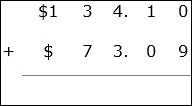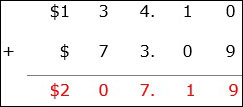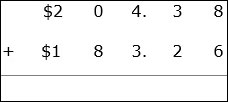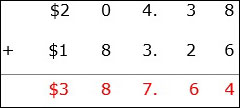Addition of money in dollars and cents is same as the addition of decimal numbers.

• The money amounts to be added are lined up vertically with the decimal points and digits as per place values aligned one above the other.

• The digits in the rightmost column are first added.

• Then the digits of all columns are added one by one till we get the sum of the money amounts.

• We bring down the decimal point and the dollar sign at the right places.

Add $134.10 +$73.09

### Solution

Step 1:The money amounts to be added are lined up vertically with the decimal points and dollar signs aligned one above the other’

Step 2:

We begin at the right most column 0 + 9 = 9; next 1 + 0 = 1; 4 + 3 = 7;

3 + 7 = 10, we bring down 0 and carry 1 to next column; next 1 + 1 = 2.

Step 3:

So we get the final sum of the money amounts as $207.19Add$204.38 + 183.26 ### Solution Step 1:The money amounts to be added are lined up vertically with the decimal points and dollar signs aligned one above the other. Step 2: We begin at the right most column 8 + 6 = 14, bring down 1 and carry 1; next 1 + 3 + 2 = 6; Next 4 +3 = 7; 0 + 8 = 8, and lastly 2 + 1 = 3 Step 3: So we get the final sum of the money amounts as 387.64